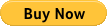## Competition Math for Elementary School

### PDF files // not hard copies

For students in grades 3-5 who:

• seek material more challenging than they typically encounter in their math classroom
• would like to build a solid problem solving foundation for future math competitions such as AMC and Mathcounts.
• Each chapter consists of:
(1) basic skill and knowledge section with plenty of examples,
(2) exercise problems,
(3) detailed solutions to all problems.

# Competition Math for Elementary School Volume 3PDF: (\$9.99)Chapter 1. Patterns with Figures1

Chapter 2. Fast Ways Addition and Subtraction 16

Chapter 3. Patterns with Numbers 33

Chapter 4. Fast Ways Multiplication 50

Chapter 5. Counting Figures 64

Chapter 6. Math Riddles: Addition and Subtraction 91

Chapter 7. Math Riddles: Multiplications and Divisions 118

Chapter 8. Magic Squares 146

Chapter 9. Perimeters 169

Index 189

# Competition Math for Elementary School Volume 4PDF: (\$9.99)Chapter 1. Pattern with Days 1

Chapter 2. Average 25

Chapter 3. Sums and Difference 48

Chapter 4. Sum and Multipliers77

Chapter 5. Difference and Multipliers 110

Chapter 6. Back Calculation 130

Chapter 7. Ratio and Rate 151

Chapter 8. Dividing with Remainders 167

Chapter 9. Chickens and Rabbits 187

Index 210